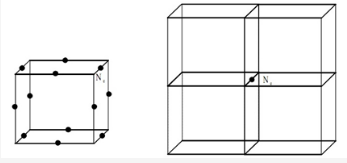# What are the no of atoms in an edge centered unit cell and how to calculate for edge center?

what are the no of atoms in an edge centered unit cell and how to calculate for edge center?

In edge centered unit cell atoms are present at corners as well as at centers of each edges.

An edge atom and edge centered is general to four unit cells and there are twelve edges of the unit cell. The contribution from each edge atom is thus 1/4 . The number of atoms per unit cell in edge centre is given by,

= (Nc/8) + (Ne/4)
= (8/8) + (12/4)
= 4

The number of atoms per unit cell in such case is four. Three from 12 edges and 1 from eight corners

Here,
Ne = Number of atoms at the edge centre
Nc = Number of corners